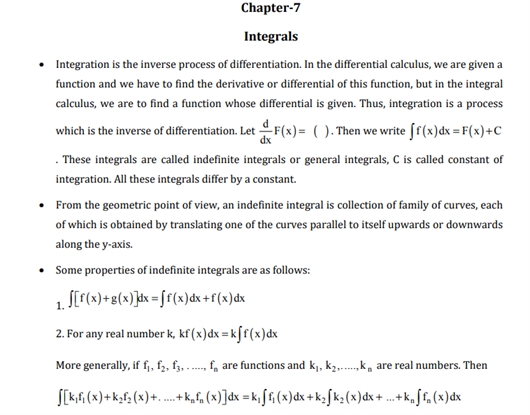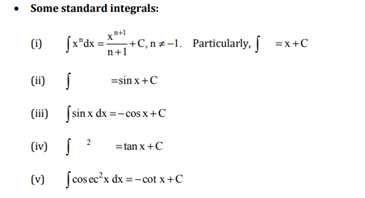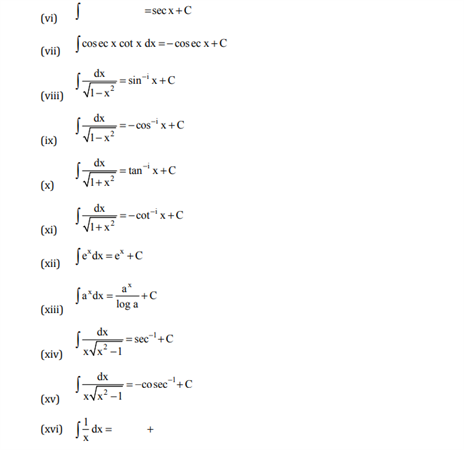# Maths Revision Notes for Class 12 Chapter 7 Integrals.

Integrals is the 7th chapter present in the CBSE Class 12 Maths revision notes. Students here learn about the definition of Integration, along with the process of finding a function for a particular integral. They can also understand different concepts such as an indefinite integral, properties of indefinite integration, standard formulas of Integration, methods of Integration, methods of substitution and some special integrals. Other major methods introduced in this chapter are methods of partial fractions for rational functions and method of integration by parts as well.

You can find the Revision notes for Integrals, available below:You may also want to read more:

 NCERT Related Articles NCERT Solutions for Class 12 NCERT Solutions for Class 11 NCERT Solutions for Class 10 NCERT Solutions for Class 12 Maths NCERT Solutions for Class 11 Maths NCERT Solutions for Class 10 Maths NCERT Solutions for Class 12 Physics NCERT Solutions for Class 11 Physics NCERT Solutions for Class 10 Science NCERT Solutions for Class 12 Chemistry NCERT Solutions for Class 11 Chemistry NCERT Solutions for Class 6 NCERT Solutions for Class 12 Biology NCERT Solutions for Class 11 Biology NCERT Solutions for Class 6 Maths NCERT Solutions for Class 9 NCERT Solutions for Class 8 NCERT Solutions for Class 6 Science NCERT Solutions for Class 9 Maths NCERT Solutions for Class 8 Maths NCERT Solutions for Class 4 NCERT Solutions for Class 9 Science NCERT Solutions for Class 8 Science NCERT Solutions for Class 4 Maths NCERT Solutions for Class 5 NCERT Solutions for Class 7 NCERT Solutions for Class 7 Maths NCERT Solutions for Class 4 Science NCERT Solutions for Class 5 Maths NCERT Solutions for Class 7 Science NCERT Solutions NCERT Books NCERT Solutions for Class 5 Science